Next: Coulomb Interaction Up: Klein-Gordon Equation Previous: Zitterbewegung

# Klein Paradox for Spin-0 Particles

We consider the scattering of a Klein-Gordon particle of energy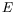and momentum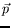from a electrostatic step-function potential as shown in figure 4.1. This problem is an archetype problem in nonrelativistic quantum mechanics. For relativistic quantum mechanics, we will find that the solution leads to a paradox (Klein paradox) when the potential is strong.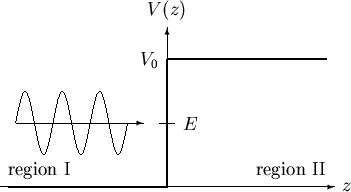Mathematically the step-function electromagnetic potential can be written as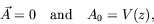(4.137)

where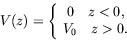(4.138)

The Klein-Gordon equation in the presence of this potential becomes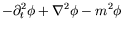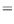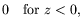(4.139)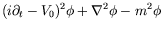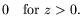(4.140)

A positive-energy incoming beam (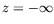) is of the form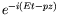. We look for solutions of the form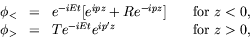(4.141)

where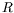and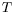are reflected and transmitted amplitudes, respectively, and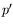is the momentum in region II. Substitution of these solutions into the Klein-Gordon equation yields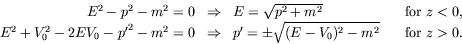(4.142)

The positive sign is chosen for the square-root when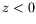, since this is imposed by our initial conditions of solving the problem for a positive-energy incoming beam. The sign for the square-root when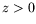can not yet be specified.

In region II there are three distinct cases, depending on the strength of the potential. This is shown in figure 4.2.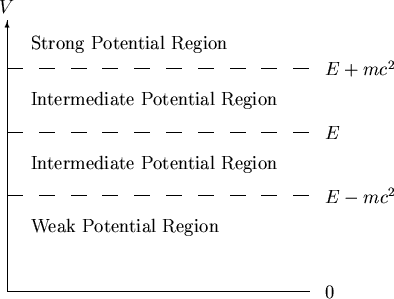weak potential: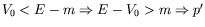is real,
intermediate potential: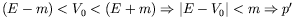is purely imaginary,
strong potential: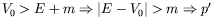is real.

We see thatcan be real or imaginary depending on the strength of the potential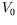. In the region of strong potential, the kinetic energy (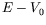) is negative, which is forbidden classically. The group velocity is given by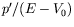and therefore has the opposite direction to the momentumin this region, if we choose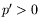. Since the group velocity is the velocity of the moving wavepacket, it looks as if the transmitted wavepacket came in from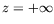; on the other hand this contradicts the condition which only allows an incoming wavepacket from. We thus have to choose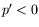(i.e. the negative sign of the square root in equation 4.142) for the case of a strong potential. Notice that this condition is not included in the Klein-Gordon equation, but is forced upon us by the physical boundary conditions.

Imposing the boundary conditions that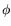and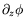be continuous at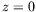gives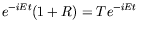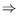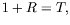(4.143)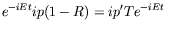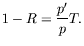(4.144)

Solving forandwe obtain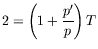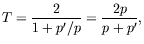(4.145)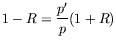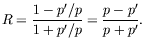(4.146)

Recall that the current is defined as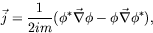(4.147)

so the incident current is thus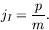(4.148)

The final currents to the left and right of the potential boundary are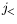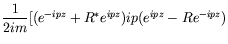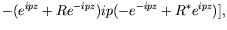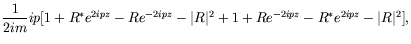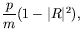(4.149)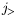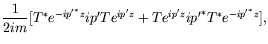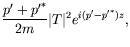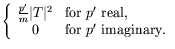(4.150)

The transmission coefficient is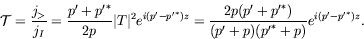(4.151)

The reflection coefficient is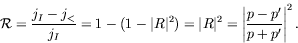(4.152)

For the case of the weak potential (real), the transmission and reflection coefficients are(4.153)

Thus the incident beam is partly reflected and partly transmitted. This is similar to the result obtained in nonrelativistic quantum mechanics. The last expression shows that the total probability is conserved.

For the case of the intermediate potential (imaginary), the transmission and reflection coefficients are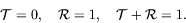(4.154)

Thus we get no transmission and only reflection. This is again the result obtained in nonrelativistic quantum mechanics and the probability is conserved.

For the case of the strong potential (real and negative), the transmission and reflection coefficients are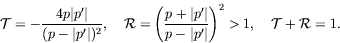(4.155)

The probability is still conserved, but only at the cost of a negative transmission coefficient and a reflection coefficient which exceeds unity. The strong potential appears to give rise to a paradox. There is no paradox if we consider that in the strong potential case the potential is strong enough to create particle-antiparticle pairs. The antiparticles are attracted by the potential and create a negative charged current moving to the right. This is the origin of the negative transmission coefficient. The particles, on the other hand, are reflected from the barrier and combined with the incident particle beam (which is completely reflected) leading to a positively charged current, moving to the left and with magnitude greater than that of the incident beam.Next: Coulomb Interaction Up: Klein-Gordon Equation Previous: Zitterbewegung
Douglas M. Gingrich (gingrich@ ualberta.ca)
2004-03-18